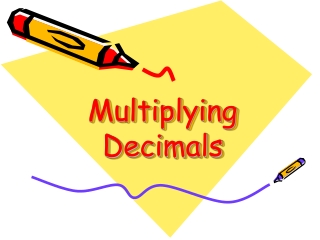DownloadDownload PresentationMultiplying Decimals

# Multiplying Decimals

Télécharger la présentation## Multiplying Decimals

- - - - - - - - - - - - - - - - - - - - - - - - - - - E N D - - - - - - - - - - - - - - - - - - - - - - - - - - -
##### Presentation Transcript

1. Multiplying Decimals

2. Example: 2.7 x 3.5

3. Step 1 Multiply as you normally would 27 x 35 945

4. Step 2 Count the number of digits behind a decimal point in the problem. This one has two digits behind the decimal point, the 7 and the 5. 2.7 x 3.5 =

5. Step 3 Put the decimal point in the answer. If your problem had 2 digits behind a decimal point, your answer should have 2 digits behind a decimal point.

8. Step 4 Add zeros if needed.

9. Let’s try a few together.

10. 7.56 x 3.8 = 287.28 2872.8 28.728

11. .3445 x 3.9 = 1.34355 13.4355 134.355

12. 13.723 x 122.5 = 16.810675 168.10675 1681.0675

13. .55 x .007 = 38.5 .00385 .0385

14. You’re correct!

15. Incorrect, try again • Remember to count the digits behind the decimal points in the problem. • You must have this many digits behind the decimal point in the answer.